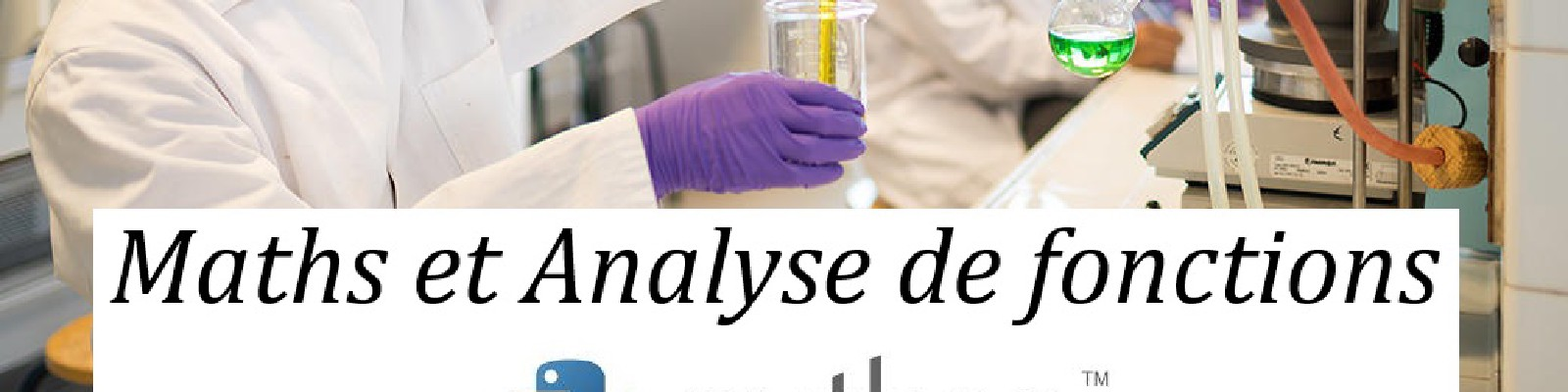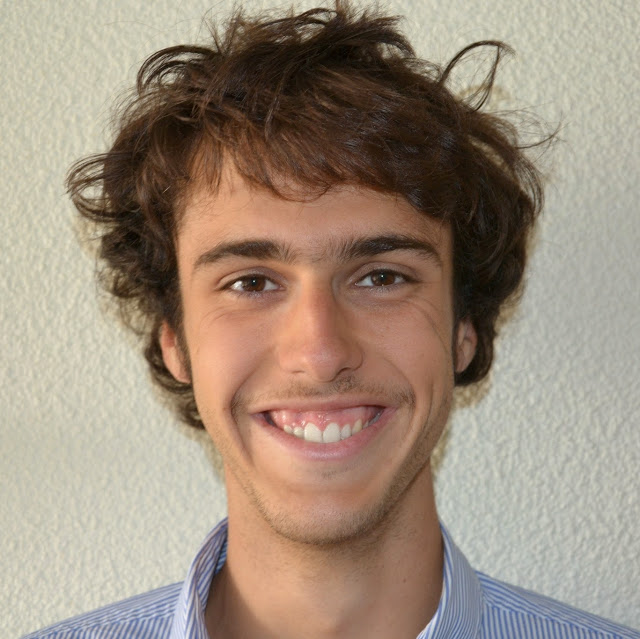Student in bio-chemistry courses © Arthur Pequin

# Overview

The Health and Science is established since 2107 and proposes to the students of second year medical studies, pharmacology and odontology an early formation to research.

# Current Course

• Introduction to noisy and uncertain models.

• Recalls on function analysis, derivation rules and extrematlity conditions. Basis functions (exponential, logarithm, trigonometric).

• Introduction to Ordinary Differential Equations (ODE).

• Case study: The fall of an apple.

# Practical work on Python

• General Introduction: The language, its main data variable structures and an example of bivariate scatterplot visualisation

• Gradient Descent: A light study of the algorithm for a real valued function of a single real variable and presentation of the performances